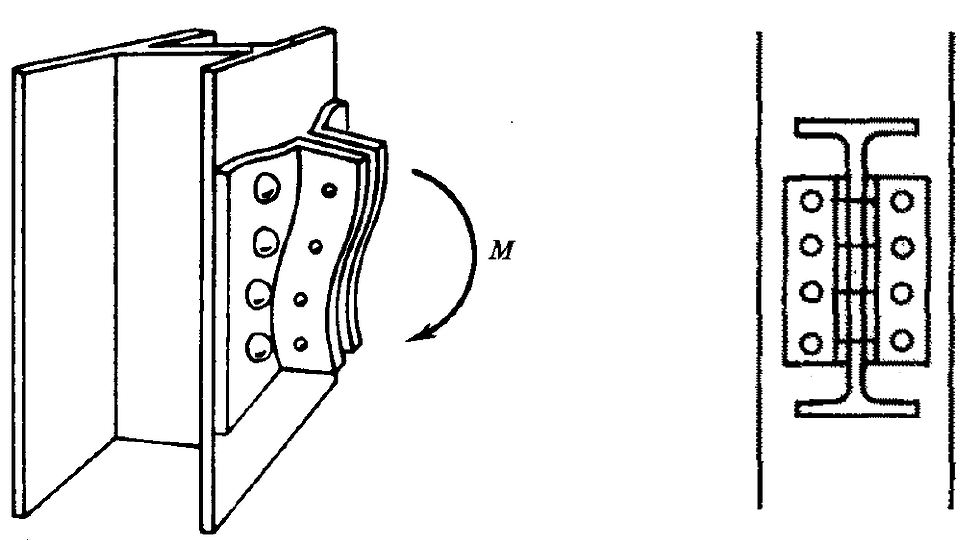top of page

# An equivalent T-stub – general explanation

The design resistance of bolted connections can be calculated using an equivalent T-stub model. This method reflects the behaviour of some elements of the connection under tension and compression and it is confirmed by test evidence.

We can calculate the capacity of the following basic components of bolted connections: end plate in bending; column flange in bending; flange cleat in bending; base plate in bending under tension; base plate in compression.

The figures below give a better understanding of the locations of equivalent T-stubs within a connection.Figure 1.

In an end plate to column flange connection, two typical T-stubs can be specified: a column flange T-stub, shown in blue, and an end plate T-stub, shown in red in Figure 1.Figure 2.

To model part of an extended end plate connection, a cleat, formed by an extended plate and part of the flange, is notionally changed to a T-stub with the length two times shorter, as shown above in Figure 2.Figure 3.

Similarly to Figure 2, we can apply the same principle to a flange angle cleat (Figure 3).Figure 4.

A web angle cleat can be directly used as a T-stub, formed of group, where it may be necessary to divide this group into separate T-stubs for each bolt row (Figure 4).Figure 5.

The design resistance of concrete in compression along with the thickness of the base plate can be calculated using a method with equivalent T-stubs for flanges and web.

For example, the design resistance of a column base plate with an I-section subject to compression only may be determined by adding together the individual resistance of two T-stubs under the column flange and one T-stub under the column web (Figure 5).Figure 6.

Base plates in bending under tension may be assessed using the same concept with equivalent T-stubs (Figure 6).

It should be noted that the effective length of equivalent T-stubs in each case is a notional length and does not necessarily correspond to the physical length of the component.

Tags: